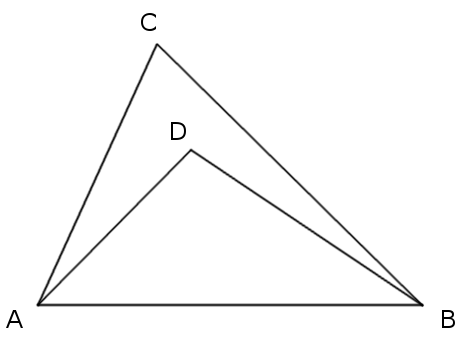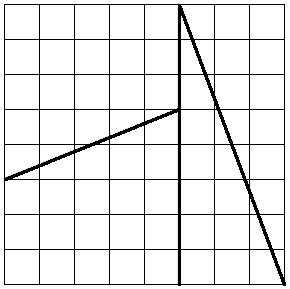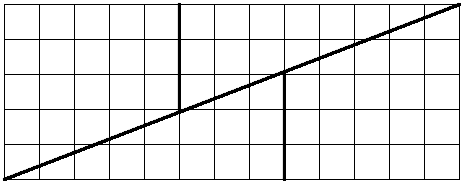# An Evening StrollMaybe this was inevitable: A team of mathematicians have worked out the most efficient pub crawl in the United Kingdom, connecting 24,727 pubs in the shortest possible closed loop, 45,495,239 meters, or about 28,269 miles. Because it’s a loop, a determined crawler can start at any point and eventually find himself back home.

Despite the pickled application, this represents a serious achievement in computational mathematics, an advance in the so-called traveling salesman problem (TSP), which asks for the shortest route that passes through each of a set of points once and once only. The pub crawl includes more than 100 times the previous record number of stops in a road-distance TSP.

“We, of course, did not have in mind to bring everything mathematics has to bear in order to improve the lot of a wandering pub aficionado,” wrote lead researcher William Cook of the University of Waterloo. “The world has limited resources and the aim of the applied mathematics fields of mathematical optimisation and operations research is to create tools to help us to use these resources as efficiently as possible.”

(Thanks, Danesh.)

Reader Alex Freuman passed this along — a simple method of establishing any row in Pascal’s triangle, attributed to Edric Cane. To establish, for example, the seventh row (after the initial solitary 1), create a row of fractions in which the numerators are 7, 6, 5, 4, 3, 2, 1 and the denominators are 1, 2, 3, 4, 5, 6, 7:$\displaystyle \frac{7}{1} \times \frac{6}{2} \times \frac{5}{3} \times \frac{4}{4} \times \frac{3}{5} \times \frac{2}{6} \times \frac{1}{7}$

Now multiply these in sequence, cumulatively, to get the numbers for the seventh row of the triangle:$\displaystyle 1 \quad 7 \quad 21 \quad 35 \quad 35 \quad 21 \quad 7 \quad 1$

These are the coefficients for$\displaystyle \left ( a+b \right )^{7}=a^{7} + 7a^{6}b + 21a^{5}b^{2} + 35a^{4}b^{3} + 35a^{3}b^{4} + 21a^{2}b^{5} + 7ab^{6} + b^{7}$.

Cane writes, “It couldn’t be easier to remember or to implement.” Another example — row 10:$\displaystyle \frac{10}{1} \times \frac{9}{2} \times \frac{8}{3} \times \frac{7}{4} \times \frac{6}{5} \times \frac{5}{6} \times \frac{4}{7} \times \frac{3}{8} \times \frac{2}{9} \times \frac{1}{10}$$\displaystyle 1 \quad 10 \quad 45 \quad 120 \quad 210 \quad 252 \quad 210 \quad 120 \quad 45 \quad 10 \quad 1$

# The Jeep Problem

An adventurer wants to explore a desert. He has a jeep that can carry up to 1 unit of fuel at any time and that will travel 1 unit of distance on 1 unit of fuel. As he travels he can leave any amount of the fuel that he’s carrying at any point, as a fuel dump to be picked up later. He starts from a fixed base at the edge of the desert, where there’s an unlimited supply of fuel. How far into the desert can he go if he wants to return safely to the base at the end of each trip?

Surprisingly, with some intelligent planning he can go as far as he likes. The diagram above shows how far he can get with 3 trips:

1. On the first trip he departs the base with 1 unit of fuel. He drives 1/6 unit into the desert, leaves 2/3 units of fuel at a fuel dump, and returns to the base using the remaining 1/6 unit of fuel.
2. On the second trip he leaves the base with 1 unit of fuel, drives 1/6 unit to the fuel dump, and draws 1/6 unit from the dump. Now he’s carrying 1 unit of fuel. Then he drives 1/4 unit farther into the desert and leaves 1/2 unit at a new dump. Now he has 1/4 unit of fuel remaining, which is just enough to reach the first fuel dump, where he collects another 1/6 unit of fuel and returns to base.
3. On the third trip he drives 1/6 unit to reach the first fuel dump, where he tops up with 1/6 unit of fuel (leaving 1/6 unit remaining there). Then he drives 1/4 to the second dump, where he collects 1/4 unit of fuel (again topping up to 1 full unit). (1/4 unit now remains in the second fuel dump.) Now he can drive 1/2 unit distance into the desert before he has to return to the second fuel dump, where he collects the remaining 1/4 unit fuel, which enables him to reach the first fuel dump, where he collects the last 1/6 unit of fuel, which is just enough to get back to base.

So if 3 trips are planned the explorer can travel a round-trip distance of 1 + 1/2 + 1/3 = 11/6 units. You can see the pattern: If the explorer had planned 4 trips, he would set up fuel dumps at distances of 1/8, 1/6, and 1/4 from the base, initially storing 3/4, 2/3, and 1/2 units at each and then drawing 1/8, 1/6, and 1/4 units of fuel from each on each visit. As before, on the final trip he could depart the last fuel dump with a full tank, drive 1/2 unit into the desert, and then return to the base, exhausting each fuel dump on the way. In that case he’d have traveled a round-trip distance of 1 + 1/2 + 1/3 + 1/4 = 25/12 units.

This is just the harmonic series, 1 + 1/2 + 1/3 + 1/4 + 1/5 + …, which is divergent — in principle, at least, the explorer can travel as far as he likes into the desert, provided he plans a large enough series of trips. In practice it would be very difficult, though — both the number of fuel dumps and the total amount of fuel necessary increase exponentially with the distance to be traveled.

# Solitons

In 1834, engineer John Scott Russell was experimenting with boats in Scotland’s Union Canal when he made a strange discovery:

I was observing the motion of a boat which was rapidly drawn along a narrow channel by a pair of horses, when the boat suddenly stopped — not so the mass of water in the channel which it had put in motion; it accumulated round the prow of the vessel in a state of violent agitation, then suddenly leaving it behind, rolled forward with great velocity, assuming the form of a large solitary elevation, a rounded, smooth and well-defined heap of water, which continued its course along the channel apparently without change of form or diminution of speed. I followed it on horseback, and overtook it still rolling on at a rate of some eight or nine miles an hour, preserving its original figure some thirty feet long and a foot to a foot and a half in height. Its height gradually diminished, and after a chase of one or two miles I lost it in the windings of the channel. Such, in the month of August 1834, was my first chance interview with that singular and beautiful phenomenon which I have called the Wave of Translation.

They’re known today as solitons. He found that such waves can travel over very large distances, at a speed that depends on their size and width and the depth of the water. Remarkably, as shown above, they emerge from a collision unchanged, simply “passing through” one another.

(Thanks, Steve.)

# Kapitza’s Pendulum

Here’s a surprise: A pendulum can be made stable in its inverted position if its support is oscillated rapidly up and down.

Even more improbably, in 1993 David Acheson and Tom Mullin showed that a double and even a triple pendulum can be made to stand up vertically like this if the pivot vibrates at the right frequency.

“The ‘trick’ really did work, and it worked, in fact, far better than we could ever have imagined,” Acheson wrote in 1089 and All That. “We were quite taken aback by just how stable the inverted state could be, and provided the pendulums were kept roughly aligned with one another, we could push them over by as much as 40 degrees or so and they would still gradually wobble back to the upward vertical.”

They published their results in Nature and later appeared on the BBC program Tomorrow’s World, but their demonstration didn’t impress everyone — afterward, an outraged caller upbraided the producers for “lowering their usually high standards” and “falling prey to two tricksters from Oxford.”

Here’s the double pendulum (thanks, Eccles):

# Fence Work

In 1951, Arthur B. Brown of Queens College noted that the number 3 can be expressed as the sum of one or more positive integers in four ways (taking the order of terms into account):

3
1 + 2
2 + 1
1 + 1 + 1

As it turns out, any positive integer n can be so expressed in 2n – 1 ways. Brown asked, how can this be proved?

William Moser of the University of Toronto offered this insightful solution:

Imagine the digit 1 written n times in a row. For example, if n = 4:

1 1 1 1

This is a picket fence, with n pickets and n – 1 spaces between them. At each space we can choose either to insert a plus sign or leave it blank. So that gives us n – 1 tasks to perform (i.e., making this choice for each space) and two options for each choice. Thus the total number of expressions for n as a sum is 2n – 1, or, in the case of n = 4, eight:

1 1 1 1 = 4
1 + 1 1 1 = 1 + 3
1 1 + 1 1 = 2 + 2
1 1 1 + 1 = 3 + 1
1 + 1 + 1 1 = 1 + 1 + 2
1 + 1 1 + 1 = 1 + 2 + 1
1 1 + 1 + 1 = 2 + 1 + 1
1 + 1 + 1 + 1 = 1 + 1 + 1 + 1

(Pi Mu Epsilon Journal 1:5 [November 1951], 186.)

# First and Last

The fifth power of any one-digit number ends with that number:

05 = 0
15 = 1
25 = 32
35 = 243
45 = 1024
55 = 3125
65 = 7776
75 = 16807
85 = 32768
95 = 59049

11/26/2016: UPDATE, after hearing from some readers who are thinking more deeply than I am:

First, this immediately implies that any integer raised to the fifth power ends with the same digit as the original number.

Second, the same effect occurs regularly at higher powers, specifically 9, 13, 17, and x = 1 + 4n where n = {0, 1, 2, 3, …}.

Does anyone know what this rule is called? I found it in Reuben Hersh and Vera John-Steiner’s 2011 book Loving + Hating Mathematics — Eugene Wigner writes of falling in love with numbers at his school in Budapest: “After a few years in the gymnasium I noticed what mathematicians call the Rule of Fifth Powers: That the fifth power of any one-digit number ends with that same number. Thus, 2 to the fifth power is 32, 3 to the fifth power is 243, and so on. At first I had no idea that this phenomenon was called the Rule of Fifth Powers; nor could I see why it should be true. But I saw that it was true, and I was enchanted.”

I actually can’t find a rule by that name. Perhaps it goes by a different name in English-speaking countries?

12/08/2016 UPDATE: It’s a consequence of Fermat’s little theorem, as explained in this extraordinarily helpful PDF by reader Stijn van Dongen.

(Thanks to Evan, Dave, Sid, and Stijn.)

# Midnight Oil

In 1960, MIT mathematician George B. Thomas Jr. received a letter from a waterfowl farmer in Maine. The farmer thought he had discovered an error in a problem in Thomas’ influential textbook Calculus and Analytic Geometry. A little bewildered, Thomas looked into it and discovered that there was indeed an error. He thanked the writer and promised to correct the mistake in future editions.

The two corresponded intermittently thereafter, but four years went by before Thomas realized that the farmer was novelist Henry Roth, author of Call It Sleep. Suffering a disastrous case of writer’s block, Roth had turned to farming and tutoring to support his family, and he had worked his way through every problem in Thomas’ book, ninety per chapter, “often struggling long into the night before arriving at the solution,” according to biographer Steven Kellman.

A copy of the textbook, “inscribed with notes,” is listed among Roth’s papers. In the preface to the fourth edition, Thomas wrote, “One of the author’s friends, Mr. Henry Roth, wrote that he feared that the new edition would be ‘rife with set theory.’ I believe that he, and others who have used the third edition, will find that only modest additions of set theory have been made.”

# Point to PointHere’s a triangle, ABC, and an arbitrary point, D, in its interior. How can we prove that AD + DB < AC + CB?

The fact seems obvious, but when the problem is presented on its own, outside of a textbook or some course of study, we have no hint as to what technique to use to prove it. Construct an equation? Apply the Pythagorean theorem?

“The issue is more serious than it first appears,” write Zbigniew Michalewicz and David B. Fogel in How to Solve It (2000). “We have given this very problem to many people, including undergraduate and graduate students, and even full professors in mathematics, engineering, or computer science. Fewer than five percent of them solved this problem within an hour, many of them required several hours, and we witnessed some failures as well.”

Here’s a dismaying hint: Michalewicz and Fogel found the problem in a math text for fifth graders in the United States. What’s the answer?

# Shifting AreasThis square of 8 × 8 = 64 square units can apparently be reassembled into a rectangle of 5 × 13 = 65 square units:This paradox is described in W.W. Rouse Ball’s 1892 Mathematical Recreations and Essays; it seems to have been published first in 1868 in Zeitschrift für Mathematik und Physik.

In 1938 the Rockefeller Foundation’s Warren Weaver discovered an old trove of papers from the 1890s in which Lewis Carroll puzzled out the dimensions of all possible squares in which this illusion is possible (the other sizes include squares of 21 and 55 units on a side).

Regardless of publication, it’s not clear who first came up with the idea. Sam Loyd claimed to have presented it to the American Chess Congress in 1858. That would be interesting, as it was his son who later discovered that the four pieces can be assembled into a figure of 63 squares:(Warren Weaver, “Lewis Carroll and a Geometrical Paradox,” American Mathematical Monthly 45:4 [April 1938], 234-236.)